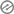# Mathematics Primary 6 First Term

Edu Delight Tutors

FIRST TERM EXAMINATION 2020/2021

CLASS: PRIMARY 6 SUBJECT: MATHEMATICSNAME:……………………………………………………………………………………1.) Write One million, one hundred thousand and one in figures

(a) 1001001 (b) 1100001 (c) 1010010

2.) Write out all factors of 8 (a) 1, 2, 3, 4 (b) 1, 2, 4, 8 (c) 8, 16, 24, 323.) Circle the one that is not a prime number 3, 13, 33 (a) 3 (b) 13 (c) 33

4.) Circle the one that is a prime number 4, 1, 3 (a) 4 (b) 1 (c) 3

5.) What is the place value of 8 in 12.841 (a) 8thousand (b) 8tenths (c) 8units6.) Write 1999 in Roman figures (a) MCMXCIX (b) MMCXIX (c) MMIXCIX

7.) Express 35/100 as a fraction in its lowest term (a) 7/20 (b) 3/10 (c) 5/20

8.) Express 31/7 as an improper fraction (a) 31/7 (b) 22/7 (c) 13/79.) Convert ¼ to decimal (a) 1.4 (b) 0.25 (c) 0.14

10.) Add up 0.48 and 31.2 (a) 31.68 (b) 31.248 (c) 0.4831Section B: Show all Workings

1.) Write out all factors of 12

b.) Write out all factors of 322.) Express the prime factors of 32 in index form

b.) Express the prime factors of 90 in index form

3.) Calculate the HCF of 12 and 24b.) Calculate the HCF of 8 and 40